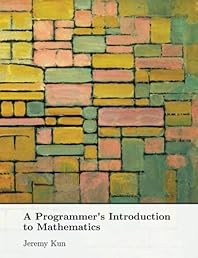A Programmer's Introduction to Mathematics Dr. Jeremy Kun

November 27th, 2018

Price: \$35.00

(as of 2018-12-02 08:00:28 PST)

Usually ships in 24 hours

Literature & Fiction

Released: 2018-11-27

A Programmer's Introduction to Mathematics by Dr. Jeremy Kun

Description

A Programmer's Introduction to Mathematics uses your familiarity with ideas from programming and software to teach mathematics. You'll learn about the central objects and theorems of mathematics, including graphs, calculus, linear algebra, eigenvalues, optimization, and more. You'll also be immersed in the often unspoken cultural attitudes of mathematics, learning both how to read and write proofs while understanding why mathematics is the way it is. Between each technical chapter is an essay describing a different aspect of mathematical culture, and discussions of the insights and meta-insights that constitute mathematical intuition. As you learn, we'll use new mathematical ideas to create wondrous programs, from cryptographic schemes to neural networks to hyperbolic tessellations. Each chapter also contains a set of exercises that have you actively explore mathematical topics on your own. In short, this book will teach you to engage with mathematics. A Programmer's Introduction to Mathematics is written by Jeremy Kun, who has been writing about math and programming for 8 years on his blog “Math Intersect Programming.” As of 2018, he works in datacenter optimization at Google.

Book Details

Author: Dr. Jeremy Kun Publisher: CreateSpace Indepe.. Binding: Paperback Language: English Pages: 378Content RSSComments RSS